Publicité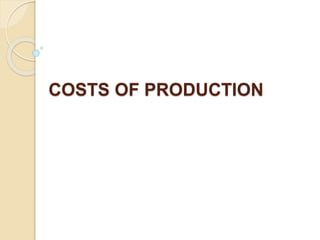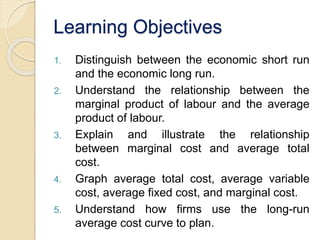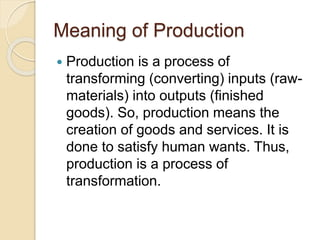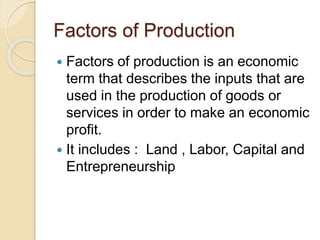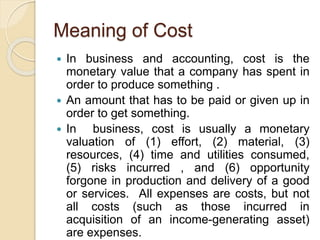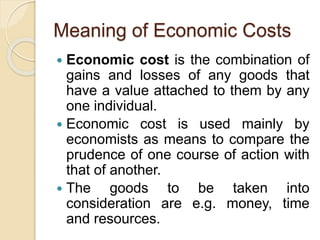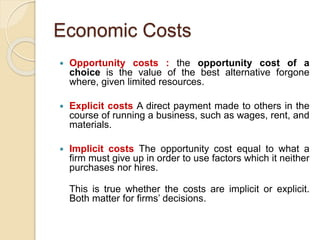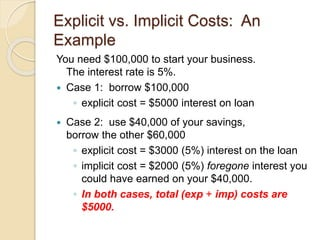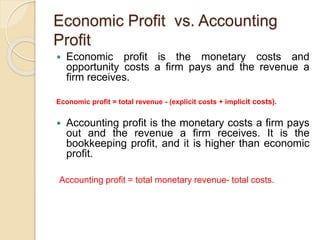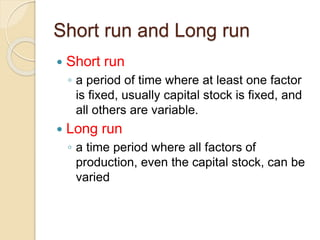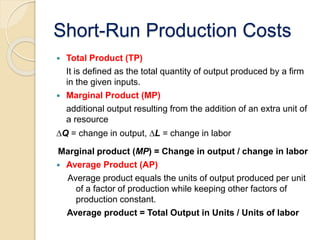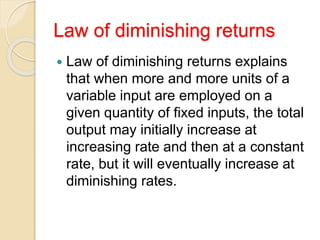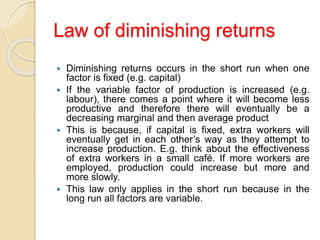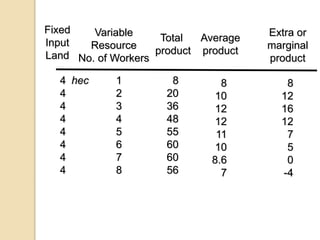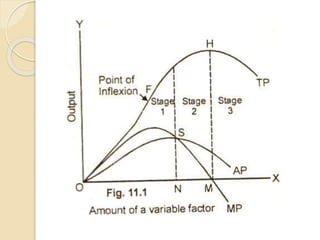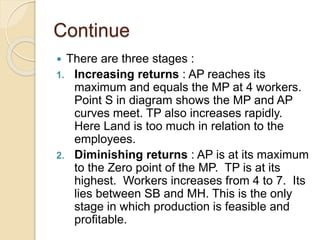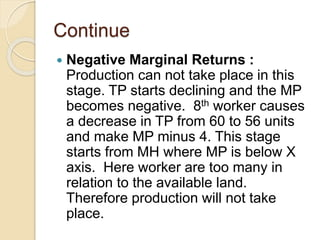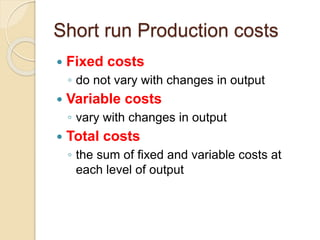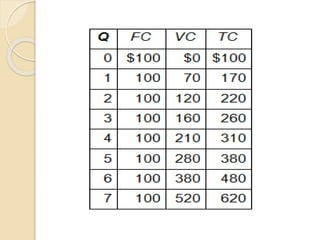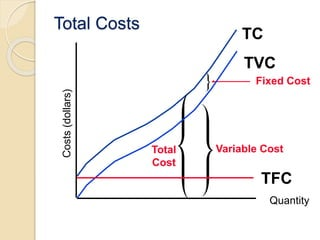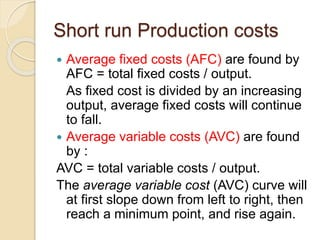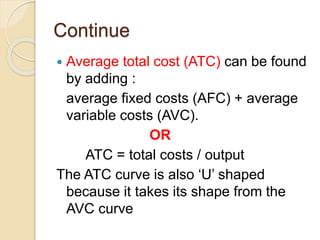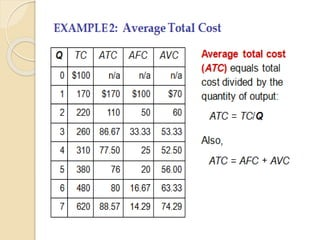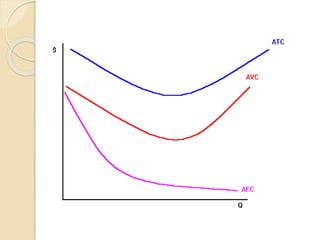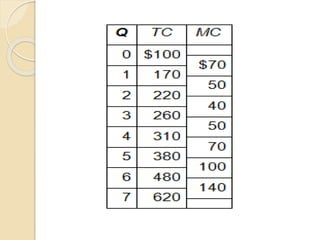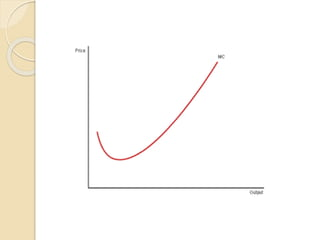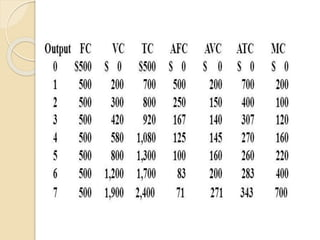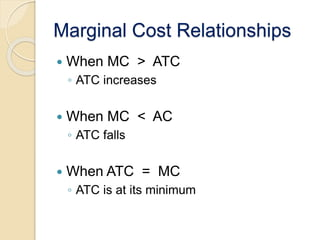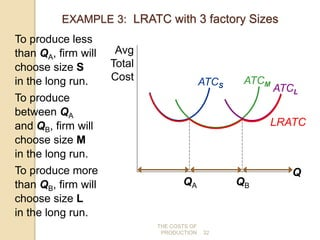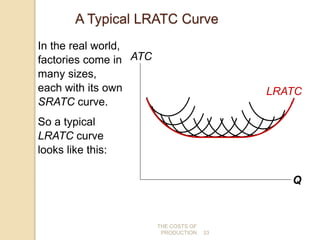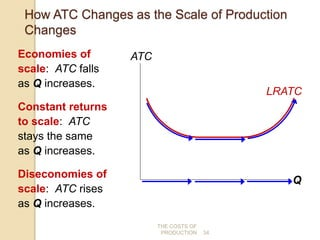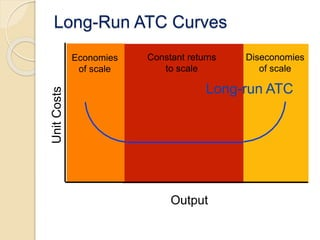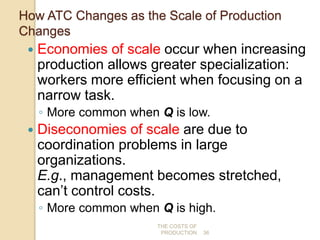1 sur 36
Publicité

### Costs of Production

1. COSTS OF PRODUCTION
2. Learning Objectives 1. Distinguish between the economic short run and the economic long run. 2. Understand the relationship between the marginal product of labour and the average product of labour. 3. Explain and illustrate the relationship between marginal cost and average total cost. 4. Graph average total cost, average variable cost, average fixed cost, and marginal cost. 5. Understand how firms use the long-run average cost curve to plan.
3. Meaning of Production  Production is a process of transforming (converting) inputs (raw- materials) into outputs (finished goods). So, production means the creation of goods and services. It is done to satisfy human wants. Thus, production is a process of transformation.
4. Factors of Production  Factors of production is an economic term that describes the inputs that are used in the production of goods or services in order to make an economic profit.  It includes : Land , Labor, Capital and Entrepreneurship
5. Meaning of Cost  In business and accounting, cost is the monetary value that a company has spent in order to produce something .  An amount that has to be paid or given up in order to get something.  In business, cost is usually a monetary valuation of (1) effort, (2) material, (3) resources, (4) time and utilities consumed, (5) risks incurred , and (6) opportunity forgone in production and delivery of a good or services. All expenses are costs, but not all costs (such as those incurred in acquisition of an income-generating asset) are expenses.
6. Meaning of Economic Costs  Economic cost is the combination of gains and losses of any goods that have a value attached to them by any one individual.  Economic cost is used mainly by economists as means to compare the prudence of one course of action with that of another.  The goods to be taken into consideration are e.g. money, time and resources.
7. Economic Costs  Opportunity costs : the opportunity cost of a choice is the value of the best alternative forgone where, given limited resources.  Explicit costs A direct payment made to others in the course of running a business, such as wages, rent, and materials.  Implicit costs The opportunity cost equal to what a firm must give up in order to use factors which it neither purchases nor hires. This is true whether the costs are implicit or explicit. Both matter for firms’ decisions.
8. Explicit vs. Implicit Costs: An Example You need \$100,000 to start your business. The interest rate is 5%.  Case 1: borrow \$100,000 ◦ explicit cost = \$5000 interest on loan  Case 2: use \$40,000 of your savings, borrow the other \$60,000 ◦ explicit cost = \$3000 (5%) interest on the loan ◦ implicit cost = \$2000 (5%) foregone interest you could have earned on your \$40,000. ◦ In both cases, total (exp + imp) costs are \$5000.
9. Economic Profit vs. Accounting Profit  Economic profit is the monetary costs and opportunity costs a firm pays and the revenue a firm receives. Economic profit = total revenue - (explicit costs + implicit costs).  Accounting profit is the monetary costs a firm pays out and the revenue a firm receives. It is the bookkeeping profit, and it is higher than economic profit. Accounting profit = total monetary revenue- total costs.
10. Short run and Long run  Short run ◦ a period of time where at least one factor is fixed, usually capital stock is fixed, and all others are variable.  Long run ◦ a time period where all factors of production, even the capital stock, can be varied
11. Short-Run Production Costs  Total Product (TP) It is defined as the total quantity of output produced by a firm in the given inputs.  Marginal Product (MP) additional output resulting from the addition of an extra unit of a resource ∆Q = change in output, ∆L = change in labor Marginal product (MP) = Change in output / change in labor  Average Product (AP) Average product equals the units of output produced per unit of a factor of production while keeping other factors of production constant. Average product = Total Output in Units / Units of labor
12. Law of diminishing returns  Law of diminishing returns explains that when more and more units of a variable input are employed on a given quantity of fixed inputs, the total output may initially increase at increasing rate and then at a constant rate, but it will eventually increase at diminishing rates.
13. Law of diminishing returns  Diminishing returns occurs in the short run when one factor is fixed (e.g. capital)  If the variable factor of production is increased (e.g. labour), there comes a point where it will become less productive and therefore there will eventually be a decreasing marginal and then average product  This is because, if capital is fixed, extra workers will eventually get in each other’s way as they attempt to increase production. E.g. think about the effectiveness of extra workers in a small café. If more workers are employed, production could increase but more and more slowly.  This law only applies in the short run because in the long run all factors are variable.
14. 1 2 3 4 5 6 7 8 8 20 36 48 55 60 60 56 8 12 16 12 7 5 0 -4 Variable Resource No. of Workers Extra or marginal product Average product Total product 8 10 12 12 11 10 8.6 7 Fixed Input Land 4 4 4 4 4 4 4 4 hec
15. Continue  There are three stages : 1. Increasing returns : AP reaches its maximum and equals the MP at 4 workers. Point S in diagram shows the MP and AP curves meet. TP also increases rapidly. Here Land is too much in relation to the employees. 2. Diminishing returns : AP is at its maximum to the Zero point of the MP. TP is at its highest. Workers increases from 4 to 7. Its lies between SB and MH. This is the only stage in which production is feasible and profitable.
16. Continue  Negative Marginal Returns : Production can not take place in this stage. TP starts declining and the MP becomes negative. 8th worker causes a decrease in TP from 60 to 56 units and make MP minus 4. This stage starts from MH where MP is below X axis. Here worker are too many in relation to the available land. Therefore production will not take place.
17. Short run Production costs  Fixed costs ◦ do not vary with changes in output  Variable costs ◦ vary with changes in output  Total costs ◦ the sum of fixed and variable costs at each level of output
18. Total Costs Quantity Costs(dollars) TC Total Cost Fixed Cost TVC Variable Cost TFC
19. Short run Production costs  Average fixed costs (AFC) are found by AFC = total fixed costs / output. As fixed cost is divided by an increasing output, average fixed costs will continue to fall.  Average variable costs (AVC) are found by : AVC = total variable costs / output. The average variable cost (AVC) curve will at first slope down from left to right, then reach a minimum point, and rise again.
20. Continue  Average total cost (ATC) can be found by adding : average fixed costs (AFC) + average variable costs (AVC). OR ATC = total costs / output The ATC curve is also ‘U’ shaped because it takes its shape from the AVC curve
21. Marginal Costs  Marginal Cost (MC) ◦ the extra, or additional cost of producing one more unit of output Marginal Cost = Change in Total Costs Change in Quantity
22. Marginal Cost Relationships  When MC > ATC ◦ ATC increases  When MC < AC ◦ ATC falls  When ATC = MC ◦ ATC is at its minimum
23. Costs in the Short Run & Long Run  Short run: Some inputs are fixed (e.g., factories, land). The costs of these inputs are FC.  Long run: All inputs are variable (e.g., firms can build more factories, or sell existing ones).  In the long run, ATC at any Q is cost per unit using the most efficient mix of inputs for that Q (e.g., the factory size with the lowest ATC).
24. THE COSTS OF PRODUCTION 31 EXAMPLE 3: LRATC with 3 factory Sizes ATCS ATCM ATCL Q Avg Total Cost Firm can choose from 3 factory sizes: S, M, L. Each size has its own SRATC curve. The firm can change to a different factory size in the long run, but not in the short run.
25. THE COSTS OF PRODUCTION 32 EXAMPLE 3: LRATC with 3 factory Sizes ATCS ATCM ATCL Q Avg Total Cost QA QB LRATC To produce less than QA, firm will choose size S in the long run. To produce between QA and QB, firm will choose size M in the long run. To produce more than QB, firm will choose size L in the long run.
26. THE COSTS OF PRODUCTION 33 A Typical LRATC Curve Q ATC In the real world, factories come in many sizes, each with its own SRATC curve. So a typical LRATC curve looks like this: LRATC
27. THE COSTS OF PRODUCTION 34 How ATC Changes as the Scale of Production Changes Economies of scale: ATC falls as Q increases. Constant returns to scale: ATC stays the same as Q increases. Diseconomies of scale: ATC rises as Q increases. LRATC Q ATC
28. Diseconomies of scale Constant returns to scale Economies of scale Long-Run ATC Curves UnitCosts Output Long-run ATC
29. THE COSTS OF PRODUCTION 36 How ATC Changes as the Scale of Production Changes  Economies of scale occur when increasing production allows greater specialization: workers more efficient when focusing on a narrow task. ◦ More common when Q is low.  Diseconomies of scale are due to coordination problems in large organizations. E.g., management becomes stretched, can’t control costs. ◦ More common when Q is high.

1. 30
2. 31
3. 32
4. 33
5. 34
6. 36
Publicité